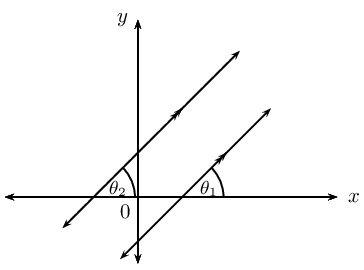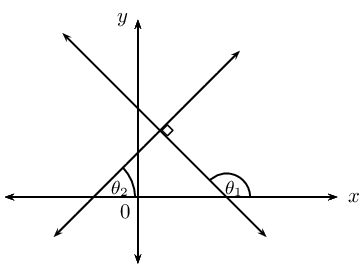Home Practice
For learners and parents For teachers and schools
Textbooks
Full catalogue
Pricing SupportLog in

We think you are located in United States. Is this correct?

# 7.4 Summary

## 7.4 Summary (EMCHX)Theorem of Pythagoras: $$AB^2 = AC^2 + BC^2$$ Distance formula: $$AB = \sqrt{(x_2 - x_1)^2 + (y_2 - y_1)^2}$$ Gradient: $$m_{AB} = \frac{y_2 - y_1}{x_2 - x_1} \quad \text{ or } \quad m_{AB} = \frac{y_1 - y_2}{x_1 - x_2}$$ Mid-point of a line segment: $$M(x;y) = \left( \frac{x_1 + x_2}{2}; \frac{y_1 + y_2}{2} \right)$$ Points on a straight line: $$m_{AB} = m_{AM} = m_{MB}$$
 Straight line equations Formulae Two-point form: $$\dfrac{y - y_1}{x - x_1} = \dfrac{y_2 - y_1}{x_2 - x_1}$$ Gradient-point form: $$y - y_1 = m (x - x_{1})$$ Gradient-intercept form: $$y = mx + c$$ Horizontal lines: $$y = k$$ Vertical lines $$x = k$$
 Parallel lines$$m_1 = m_2$$ $$\theta_1 = \theta_2$$ Perpendicular lines$$m_1 \times m_2 = -1$$ $$\theta_{1} = \text{90} ° + \theta_{2}$$
• Inclination of a straight line: the gradient of a straight line is equal to the tangent of the angle formed between the line and the positive direction of the $$x$$-axis.

$m = \tan \theta \qquad \text{ for } \text{0}° \leq \theta < \text{180}°$

• Equation of a circle with centre at the origin:

If $$P(x;y)$$ is a point on a circle with centre $$O(0;0)$$ and radius $$r$$, then the equation of the circle is:

$x^{2} + y^{2} = r^{2}$
• General equation of a circle with centre at $$(a;b)$$:

If $$P(x;y)$$ is a point on a circle with centre $$C(a;b)$$ and radius $$r$$, then the equation of the circle is:

$(x - a)^{2} + (y - b)^{2} = r^{2}$
• A tangent is a straight line that touches the circumference of a circle at only one point.

• The radius of a circle is perpendicular to the tangent at the point of contact.

temp text